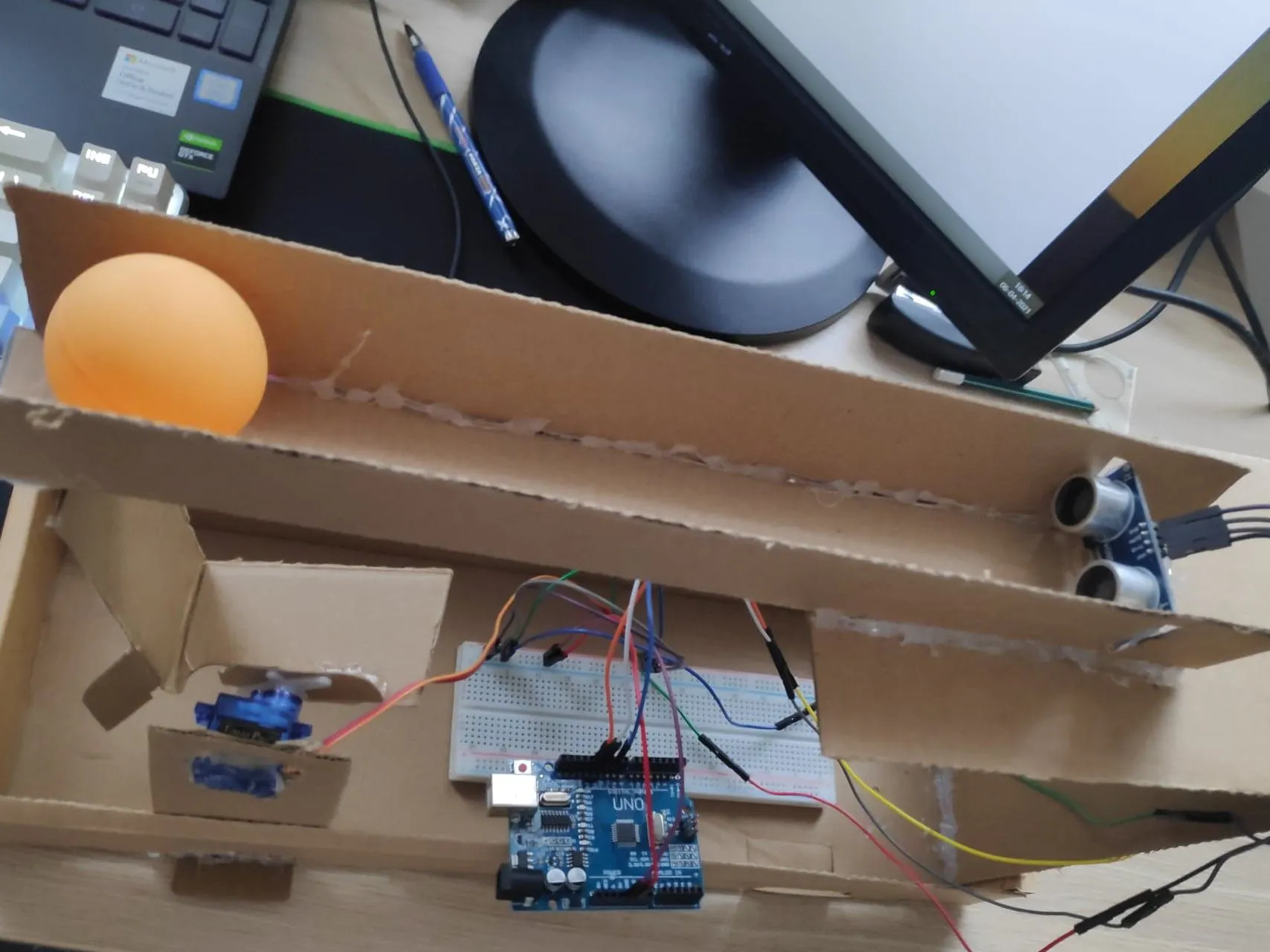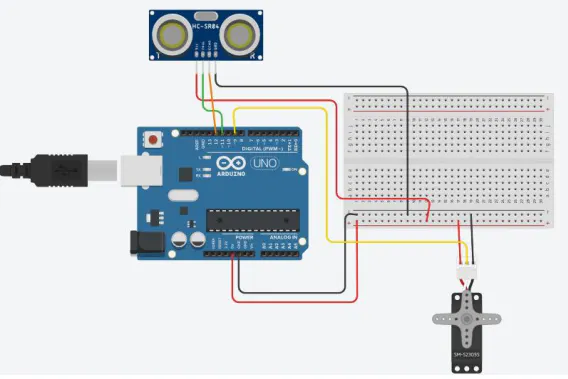Published

# Self Balancing Ball and Beam using PID control

By implementing PID control we have made the ball balance on the beam at the desired setpoint of 15 cms(total beam length = 30 cms)

BeginnerFull instructions provided154## Schematics

### schematic## Code

### Code for 30 cm beam with setpoint at 15 cm

C/C++
```#include<Servo.h>
#include<PID_v1.h>
#include<SoftwareSerial.h>

int set=6,neg=-24,pos=24,base=130;//Setpoint,Negative,Positive,Base values.neg shows tilt on other side of ultrasonic sensor
long cm1=set;                     //For filtering purpose
const double a=0.5;               //Exponential filter parameter
const int servoPin = 9;           //Servo pin

float Kp = 0.8;                                                    //Initial Proportional Gain
float Ki = 0.02;                                                      //Initial Integral Gain
float Kd = 0.75;                                                    //Intitial Derivative Gain
double Setpoint, Input, Output, ServoOutput;

PID myPID(&Input, &Output, &Setpoint, Kp, Ki, Kd, REVERSE);           //Initialize PID object, which is in the class PID.

Servo myServo;                                                       //Initialize Servo.

void setup() {

Serial.begin(9600);                                                //Begin Serial
myServo.attach(servoPin);                                          //Attach Servo

//  position as the input to the PID algorithm

myPID.SetMode(AUTOMATIC);                                         //Set PID object myPID to AUTOMATIC
myPID.SetOutputLimits(neg,pos);                                   //Set Output limits to neg and pos degrees.
}

void loop()
{

Setpoint = set;                                                      //Give value for setpoint

myPID.Compute();                                                   //computes Output in range of neg to pos degrees

ServoOutput=base+Output;                                            // value in base is my horizontal
myServo.write(ServoOutput);                                        //Writes value of Output to servo

}

delay(40);

const int TrigPin = 11;//Trig
const int EchoPin = 12;//Echo

long duration, cm,cmn;
unsigned long now = millis();
pinMode(TrigPin, OUTPUT);
digitalWrite(TrigPin, LOW);
delayMicroseconds(2);
digitalWrite(TrigPin, HIGH);
delayMicroseconds(5);
digitalWrite(TrigPin, LOW);

pinMode(EchoPin, INPUT);
duration = pulseIn(EchoPin, HIGH);

cm = duration/(15*2);

if(cm > 30)              // 30 cm is the maximum position for the ball
{cm=30;}                //Signal Conditioning for ultrasonic sensor

cmn = a * cm + (1 - a) * cm1;     //Exponential filter- signal conditioning
Serial.print(cm); Serial.print("\t");
Serial.println(cmn);            //cmn is filtered value
delay(10);
cm1 = cmn;                      //saved to cm1 which is used as history in exponential filter

return (cmn);                           //Returns filtered distance value in cm
}
```

## Credits

### nihalsuri

1 project • 1 follower